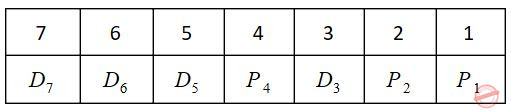## UGC NET CS 2010 Dec-Paper-2

 Question 1
The number of integers between 1 and 250 that are divisible by 2, 5 and 7 is
 A 2 B 3 C 5 D 8
Engineering-Mathematics       Combinatorics
Question 1 Explanation:
Here, we have to find all possible integers between 250 which was divisible by 2,5 and 7.
Solving this problem by 2 methods:
1. Venn diagram
2. Mathematical substitution.
In this problem, we are using Mathematical substitution method.
Step-1: According to condition, we can perform least common multiple(LCM) of 2,5 and 7=70
Step-2: Total number of integers= ⌊250/70⌋
= 3
Note: Divisible numbers are 70,140 and 210.
 Question 2
An undirected graph possesses an eulerian circuit if and only if it is connected and its vertices are
 A all of even degree B all of odd degree C of any degree D even in number
Engineering-Mathematics       Graph-Theory
Question 2 Explanation:
→ An undirected graph possesses an eulerian circuit if and only if it is connected and its vertices all of even degree.
→ Eulerian circuit (or) Euler tour in an undirected graph is a cycle that uses each edge exactly once.
 Question 3
A partially ordered set is said to be a lattice if every two elements in the set have
 A a unique least upper bound B a unique greatest lower bound C both (A) and (B) D none of the above
Engineering-Mathematics       Set-Theory
Question 3 Explanation:
A partially ordered set is said to be a lattice if every two elements in the set have
1. Unique least upper bound
2. Unique greatest lower bound
 Question 4
The minimum number of edges in a connected graph with ‘n’ vertices is equal to
 A n(n – 1) B n(n–1)/2 C n2 D n – 1
Engineering-Mathematics       Graph-Theory
Question 4 Explanation:Question 5
Consider the problem of connecting 19 lamps to a single electric outlet by using extension cords each of which has four outlets. The number of extension cords required is
 A 4 B 5 C 6 D 7
Engineering-Mathematics       Binary-Trees
 Question 6
The decimal number equivalent of (4057.06)8 is
 A 2095.75 B 2095.07 C 2095.94 D 2095.09
Digital-Logic-Design       Number-Systems
Question 6 Explanation:Question 7is equivalent to
 ABCDDigital-Logic-Design       Boolean-Expression
Question 7 Explanation:Question 8
An astable multivibrator has
 A one stable state B two stable states C no stable states D none of these
Data-Communication       multivibrator
Question 8 Explanation:Question 9
12-bit 2’s complement of –73.75 is
 A 1.001e+06 B 1.1001e+07 C 1.01101e+07 D 1.01101e+07
Digital-Logic-Design       Number-Systems
Question 9 Explanation:Question 10
Encoding of data bits 0011 into 7-bit even Parity Hamming Code is
 A 0011110 B 0101110 C 0010110 D 0011100
Digital-Logic-Design       Number-Systems
Question 10 Explanation:
(m) Data = 0011
No. of parity bits needed is decided using
2p≥ m+p+1
p=3
Bit pattern:P1(check even parity at 1, 3, 5, 7 bit) = 0
P2(check even parity at 2, 3, 6, 7 bit) = 1
P4(check even parity at 4, 5, 6, 7 bit) = 1
So encoded data = 0011110
 Question 11
How many of the following declarations are correct ?
int z = 7.0;
double void = 0.000;
short array  = {0, 1, 2};
char c = “\n”;
 A None B One is correct C Two are correct D All four are correct
Programming       Data-Type
Question 11 Explanation:
int z = 7.0; → Incorrect. Given data type is integer but given floating point number. It is wrong declaration.
double void = 0.000; → Incorrect. void is a keyword but can’t use with variable declaration.
It always return null value.
short array  = {0, 1, 2}; → Correct. It is right format. We can declare static values here itself.
char c = “\n”; → Incorrect. It is character data type but given in double quotes. It should be single quote instead of double quote.
 Question 12
The value of the following expression (13 / 4 * 3) % 5 + 1 is
 A 5.75 B 2.95 C 1.4875 D 5
Engineering-Mathematics       Combinatorics
Question 12 Explanation:
(13 / 4 * 3) % 5 + 1
Step-1: (13 / 4 * 3) % 5 + 1
Step-2: (3.25*3)%5+1
Step-3: 9.75%5+1
Step-4: 4.75+1
Step-5: 5.75
Note: ( ) having highest precedence then *,/ and % have same precedence order.
There are 12 questions to complete.

Register Now